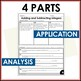# Adding and Subtracting Integers WorksheetsSubject
Resource Type
File Type

PDF

(929 KB|10 pages)
Product Rating
4.0
(1 Rating)
Standards
• Product Description
• StandardsNEW

Adding and Subtracting integers is an important skill for your 7th grade and pre algebra students. These no prep worksheets are perfect to reinforce this concept. These work great as homework or in-class work.

Each worksheet includes 4 sections: Review, Fluency, Application, and Analysis. Check out the preview for a clear understanding of how these worksheets are created.

Included in this Activity

• Each worksheet is 2 pages with 4 total sections: Review, Fluency, Application and Analysis.
• Includes two versions: A (18 questions) and B (17 questions)
• Includes a Frayer Model for the word: Integers

Other products that you may be interested in

FOLLOW ME for more great products to make sense of math!

Remember that leaving feedback earns you points toward FREE TPT purchases!

Please feel free to contact me with any questions!

Michelle,

Make Sense of Math

makesenseofmath@yahoo.com

Solve real-world and mathematical problems involving the four operations with rational numbers.
Apply properties of operations as strategies to add and subtract rational numbers.
Understand subtraction of rational numbers as adding the additive inverse, 𝘱 – 𝘲 = 𝘱 + (–𝘲). Show that the distance between two rational numbers on the number line is the absolute value of their difference, and apply this principle in real-world contexts.
Understand 𝘱 + 𝘲 as the number located a distance |𝘲| from 𝘱, in the positive or negative direction depending on whether 𝘲 is positive or negative. Show that a number and its opposite have a sum of 0 (are additive inverses). Interpret sums of rational numbers by describing real-world contexts.
Apply and extend previous understandings of addition and subtraction to add and subtract rational numbers; represent addition and subtraction on a horizontal or vertical number line diagram.
Total Pages
10 pages
Included
Teaching Duration
N/A
Report this Resource to TpT
Reported resources will be reviewed by our team. Report this resource to let us know if this resource violates TpT’s content guidelines.